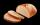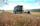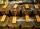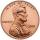# Algebra - problems - page 79

1. Surface area of the topA cylinder is three times as high as it is wide. The length of the cylinder’s diagonal is 20 cm. Find the surface area of the top of the cylinder.
2. Percentages 5\$5.25 is 7 1/2% of what number?
3. BreadsSeveral pieces of bread were in the store. Half of them plus a half of bread bought a cookbook. Of the remaining bread, half of it plus half of the bread was bought by Mr. Novák. The last bread was bought by Ms. Small. How many breads were in the store at.
4. Two forcesThe two forces F1 = 580N and F2 = 630N have the angle of 59 degrees. Calculate their resultant force F.
5. CloudsApproximately at what height is the cloud we see under an angle of 26°10' and see the Sun at an angle of 29°15' and the shade of the cloud is 92 meters away from us?
6. A squareA square with length of diagonals 12cm give: a) Calculate the area of a square b) rhombus with the same area as the square, has one diagonal with length of 16 cm. Calculate the length of the other diagonal.
7. Ľé sweetsThere are 20 sweets in the bag. Some are chocolate, other coconuts, and the remaining marzipan. Chocolate is 4 times more than coconut. Marzipan's less than chocolate. How much is in a bag of coconut sweets?
8. Find the 5Find the equation with center at (1,20) which touches the line 8x+5y-19=0
9. GardenThe garden around the new majer is divided as follows: 35% vegetable, 30% fruit orchards, 10% flowers and the remaining 120 m2 are lawns. What is the total area of the garden?
10. Ratio of sidesThe triangle has a circumference of 21 cm and the length of its sides is in a ratio of 6: 5: 3. Find the length of the longest side of the triangle in cm.
11. ProveProve that k1 and k2 is the equations of two circles. Find the equation of the line that passes through the centers of these circles. k1: x2+y2+2x+4y+1=0 k2: x2+y2-8x+6y+9=0
12. FarmlandFarmers seeded maize to 45 ha, which is 15% of their total farmland. How many hectares of fields they have?
13. Equation with xSolve the following equation: 2x- (8x + 1) - (x + 2) / 5 = 9
14. Parametric equationFind the parametric equation of a line with y-intercept (0,-4) and a slope of -2.
15. Three friendsThree friends had balls in ratio 2: 7: 4 at the start of the game. Could they have the same number of balls at the end of the game? Write 0, if not, or write the minimum number of balls they had together.
16. Tea mixtureOf the two sort of tea at a price of 180 CZK/kg and 240 CZK/kg we make a mixture 12 kg that should be prepared at a price of 200 CZK / kg. How many kilos of each sort of tea will we need to be mixed?
17. Equation with absSolve this equation with absolute value member: ?
18. Solutions, mixturesHow many liters of 70% solution we must add to 5 liters of 30% solution to give us a 60% solution?
19. DecagonCalculate the area and circumference of the regular decagon when its radius of a circle circumscribing is R = 1m
20. Cents no moreJanko bought pencils for 35 cents each. Neither he nor the salesperson had small coins just a whole € 1 coin. At least how many pencils had to buy to pay for the whole euros?

Do you have an interesting mathematical problem that you can't solve it? Enter it, and we can try to solve it.

To this e-mail address, we will reply solution; solved examples are also published here. Please enter e-mail correctly and check whether you don't have a full mailbox.